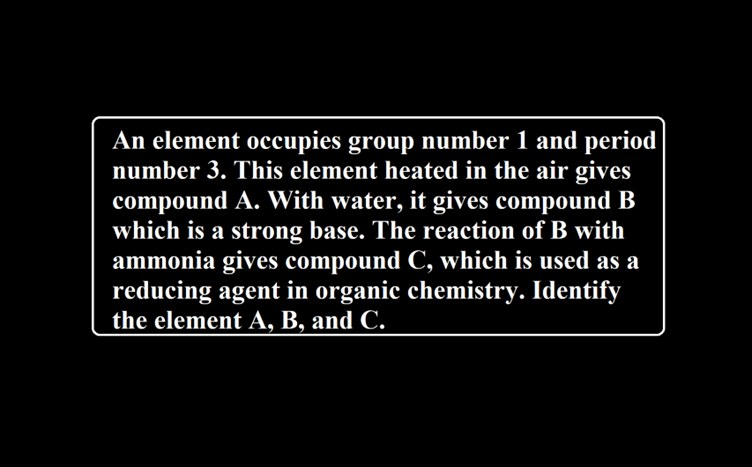# An element occupies group number 1 and period number 3. This element heated in the air gives compound A. With water, it gives compound B which is a strong base. The reaction of B with ammonia gives compound C, which is used as a reducing agent in organic chemistry. Identify the element A, B, and C.Que. An element occupies group number 1 and period number 3. This element heated in the air gives compound A. With water, it gives compound B which is a strong base. The reaction of B with ammonia gives compound C, which is used as a reducing agent in organic chemistry. Identify the element A, B, and C.
Ans. (i) As per the position in the periodic table this element occupying group number 1 and period number 3 is sodium.
(ii) When Sodium is heated in air, it forms sodium peroxide (A)
4Na + O2 → 2 Na2O2
(iii) With water, sodium gives sodium hydroxide (B)
2Na + 2H2O → 2NaOH + H2
(iv) With ammonia, sodium gives sodamide (NaNH2) at 570-670 K
2Na + 2NH3 → 2 NaNH2 + H2
The element is sodium.
Compound A is sodium peroxide.
The compound B is sodium hydroxide.
Compound C is sodamide.